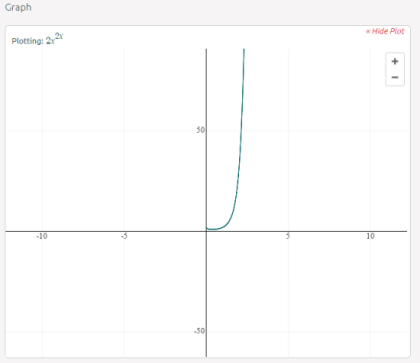Editor Ratings:
User Ratings:
[Total: 0 Average: 0]

Symbolab Math Solver is a free online math problem solver with step by step solution and graphs support. This math problem solver also displays the formula, rule, or theorem used to solve each of the mathematical problem. You can use this solver to solve mathematical problems of Algebra, Metrics & Vectors, Functions & Graphing, Trigonometry, Calculus, and Statistics.

The math solver also includes virtual keypad for typing the mathematical symbols which you don’t find on your physical keyboard. All the symbols are categorized under different categories for easier access. The solver also has an auto suggestion feature which suggests you the commonly solved mathematical problems as you start typing in the problem.## Using this Online Math Problem Solver with Complete Solution, Graphs:

To use this online math problem solver, you have to open the Symbolab Math Solver website on your browser. You will be welcomed by the homescreen of this math problem solver as shown in the screenshot below.You can see that it has the keypad containing all the mathematical symbols along with a text box. You can either type the mathematical problem or copy paste it from any other place. Just below the text box, there are a few examples of the step-by-step solution for different types of problems.

Before you proceed with entering mathematical problem and solving it, let’s take a closer look at the symbol keypad provided by the math problem solver. You can see that the symbol keypad has a total of 10 categories with each of them having corresponding symbols within it. It also has a chemistry symbol category, but it is under construction at the time of writing this article.While entering the mathematical problem, you only have to click on that specific symbol to use it in your problem. While the other things like numbers and basic mathematical operators can also be entered via physical keyboard. The cool thing about this math problem solver is that as you will start typing the problem, it starts showing suggestions.When you have entered the complete problem or chosen any of the ones from suggestions, you will be redirected to the next page known as solution page. Here, you will see the complete step-by-step solution of the problem entered by you. In the solution, some of the part may be clickable. If you will click on them then it will show you the additional details about the concept used.

At the end of the solution, you will see the graph related to then problem entered by you. You can zoom-in and zoom-out of the graph as well as drag the graph by clicking and moving the mouse.Finally in the end, the solver displays the other examples of the mathematical problems along with the option to check the web results.You may also like: 5 Free Websites to Solve Math Problems Online.

## Final Verdict:

Symbolab Math Solver is an awesome online math solver. It is one of the easiest and accurate math solver that I have ever used either online or on smartphones. I liked two things about it, the first one being the virtual keypad with all the mathematical symbols and other the auto suggestion feature. This one is must try math problem solver with no doubt.